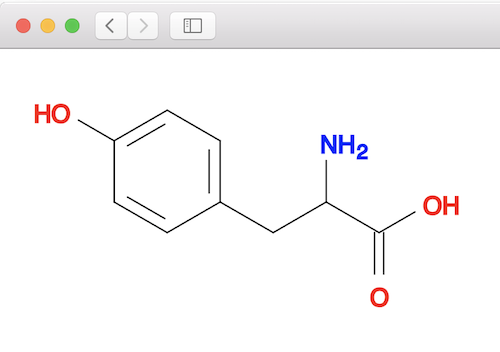Substructure Search with Wildcard Bond "~"

Q

How to use Wildcard Bond in a substructure search using "babel" commands?

✍: FYIcenter.com

AYou can use "~" in a SMARTS string as a wildcard bond to represent "ANY" bond in a substructure search using "babel" commands.

Here are some examples of substructure matching with a wildcard bond "~" in the molecule pattern:

```fyicenter\$ obabel "-:c1cc(ccc1CC(C(=O)O)N)O" -o smiles -s O-C
c1cc(ccc1CC(C(=O)O)N)O
1 molecule converted

fyicenter\$ obabel "-:c1cc(ccc1CC(C(=O)O)N)O" -o smiles -s O=C
c1cc(ccc1CC(C(=O)O)N)O
1 molecule converted

fyicenter\$ obabel "-:c1cc(ccc1CC(C(=O)O)N)O" -o smiles -s O#C
0 molecules converted

fyicenter\$ obabel "-:c1cc(ccc1CC(C(=O)O)N)O" -o smiles -s O~C
c1cc(ccc1CC(C(=O)O)N)O
1 molecule converted

fyicenter\$ obabel "-:c1cc(ccc1CC(C(=O)O)N)O" -o smiles -s O~C~C
c1cc(ccc1CC(C(=O)O)N)O
1 molecule converted

fyicenter\$ obabel "-:c1cc(ccc1CC(C(=O)O)N)O" -o smiles -s O~C~C~C
c1cc(ccc1CC(C(=O)O)N)O
1 molecule converted

fyicenter\$ obabel "-:c1cc(ccc1CC(C(=O)O)N)O" -o smiles -s O~C~C~C~c
c1cc(ccc1CC(C(=O)O)N)O
1 molecule converted

fyicenter\$ obabel "-:c1cc(ccc1CC(C(=O)O)N)O" -o smiles -s O~C~C~C~c~c
c1cc(ccc1CC(C(=O)O)N)O
1 molecule converted

fyicenter\$ obabel "-:c1cc(ccc1CC(C(=O)O)N)O" -o smiles -s O~C~C~C~c~c~c
c1cc(ccc1CC(C(=O)O)N)O
1 molecule converted

fyicenter\$ obabel "-:c1cc(ccc1CC(C(=O)O)N)O" -o smiles -s O~C~C~C~c~c~c~c
c1cc(ccc1CC(C(=O)O)N)O
1 molecule converted

fyicenter\$ obabel "-:c1cc(ccc1CC(C(=O)O)N)O" -o smiles -s O~C~C~C~c~c~c~c~O
c1cc(ccc1CC(C(=O)O)N)O
1 molecule converted
```

You can validate the above matching result by looking at the tyrosine molecule structure below:Open Babel SVG Picture - Tyrosine Molecule

2020-06-08, 843👍, 0💬